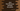# 4 different Java program to find the distances between two points## Java program to find the distances between two points:

Let’s write a Java program that finds the distance between two points. If we consider a 2-D coordinate space, we can define a point in two coordinates x and y. If we have x and y for both of the points, we can calculate the distance between them.

So, how to find the distance between two given points? What is the formula to use? Let me show you:

### Formula to find the distance between two points:

This formula is derived from the Pythagorean Theorem. If the coordinates of the points are `(x1, y1)` and `(x2, y2)`, the distance between these points can be found by using the below formula:

``√(x2 - x1)^2 + (y2 - y1)^2``

So, if we have the values of `x1, y1, x2`, and `y2`, we can find the distance between two points.

In this post, we will write one Java program that will take the values of `x1, y1, x2`, and `y2` from the user as inputs and it will find the distance between the points.

It will print the calculated distance.

We will learn two different ways to do this in Java.

### Java program 1: Find the distance between two points without any separate method:

Let’s have a look at the below program:

``````import java.util.Scanner;

public class Main {

public static void main(String[] args) {
double x1, y1, x2, y2, d;
Scanner sc = new Scanner(System.in);

System.out.println("Enter the value of x and y for the first point:");
x1 = sc.nextDouble();
y1 = sc.nextDouble();

System.out.println("Enter the value of x and y for the second point:");
x2 = sc.nextDouble();
y2 = sc.nextDouble();

d = Math.sqrt(Math.pow((x2 - x1), 2) + Math.pow((y2 - y1), 2));

System.out.println("The distance between the points is: " + d);
}
}``````

In this program,

• x1, y1, x2 and y2 are double variables to hold the coordinates of the two points. d is another double variable to hold the distance between the points.
• sc is a Scanner variable to read the user input.
• It asks the user to enter the values of x and y for both points one by one. By using the sc variable, we are reading the user input values.
• To calculate the distance between the points, we are using the Math class. sqrt method is to find the square root of the sum and pow method is to find the power.

Once the value is calculated, it prints the distance i.e. d.

If you run this program, it will print output as like below:

``````Enter the value of x and y for the first point:
1
2
Enter the value of x and y for the second point:
4
6
The distance between the points is: 5.0``````

### Method 2: By using a separate function:

We can use a separate function to do the mathematical calculation. It will take the values as parameters and return the calculated distance. Below is the complete program:

``````import java.util.Scanner;

public class Main {

private static double findDistance(double x1, double y1, double x2, double y2) {
return Math.sqrt(Math.pow((x2 - x1), 2) + Math.pow((y2 - y1), 2));
}

public static void main(String[] args) {
double x1, y1, x2, y2;
Scanner sc = new Scanner(System.in);

System.out.println("Enter the value of x and y for the first point:");
x1 = sc.nextDouble();
y1 = sc.nextDouble();

System.out.println("Enter the value of x and y for the second point:");
x2 = sc.nextDouble();
y2 = sc.nextDouble();

System.out.println("The distance between the points is: " + findDistance(x1, y1, x2, y2));
}
}``````

This program is basically same as the above one. We created a new function findDistance to calculate the distance. If you run, it will give similar output.

### Method 3: By using Math.hypot:

Math.hypot method returns `√(x^2+y^2)` without intermediate overflow or underflow. We can pass x2 - x1 and y2 - y1 as its parameters:

``````import java.util.Scanner;

public class Main {

private static double findDistance(double x1, double y1, double x2, double y2) {
return Math.hypot((x2 - x1), (y2 - y1));
}

public static void main(String[] args) {
double x1, y1, x2, y2;
Scanner sc = new Scanner(System.in);

System.out.println("Enter the value of x and y for the first point:");
x1 = sc.nextDouble();
y1 = sc.nextDouble();

System.out.println("Enter the value of x and y for the second point:");
x2 = sc.nextDouble();
y2 = sc.nextDouble();

System.out.println("The distance between the points is: " + findDistance(x1, y1, x2, y2));
}
}``````

It will give the same output.

### Method 4: By using Point2D.distance:

The distance method of Point2D class can be used to find the distance between two points in a 2D coordinate space. We need to pass the values of x1, y1, x2 and y2 to find that distance.

``````import java.awt.geom.Point2D;
import java.util.Scanner;

public class Main {

private static double findDistance(double x1, double y1, double x2, double y2) {
return Point2D.distance(x1, y1, x2, y2);
}

public static void main(String[] args) {
double x1, y1, x2, y2;
Scanner sc = new Scanner(System.in);

System.out.println("Enter the value of x and y for the first point:");
x1 = sc.nextDouble();
y1 = sc.nextDouble();

System.out.println("Enter the value of x and y for the second point:");
x2 = sc.nextDouble();
y2 = sc.nextDouble();

System.out.println("The distance between the points is: " + findDistance(x1, y1, x2, y2));
}
}``````

You will get a similar result.